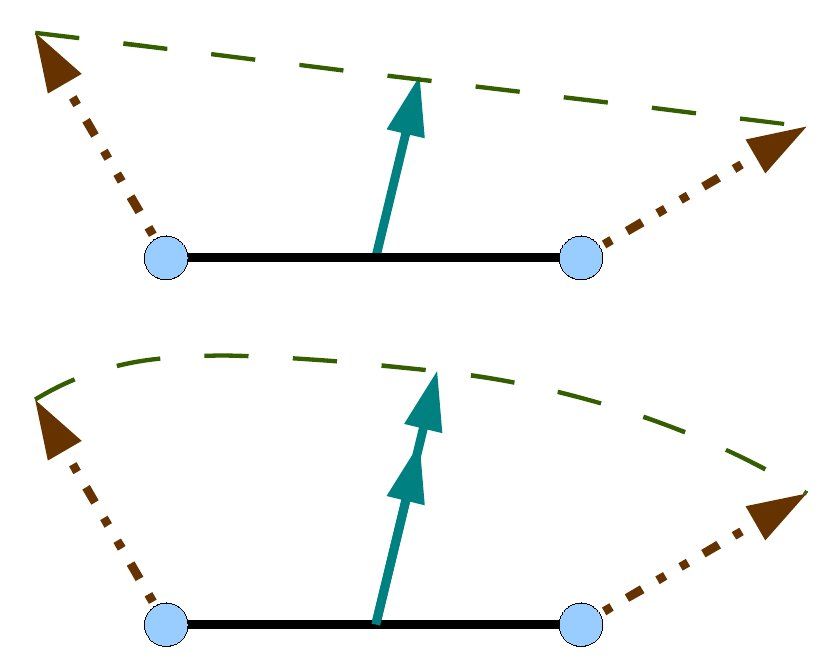Author: Romain Vergne (website)
Please cite my name and add a link to my web page if you use this course

# Image synthesis and OpenGL: lighting

## The problem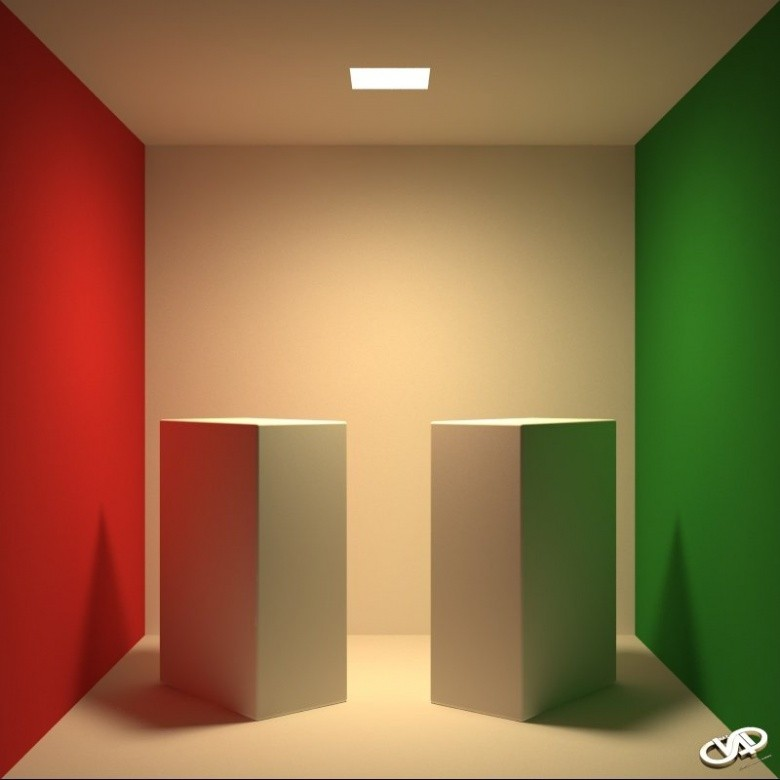The illumination on a given point of the surface depends on: primary light sources direct lighting secondary light sources all the objects in the scene an illuminated object becomes a light source indirect lighting Complex phenomenon: Color bleeding Shadows Cornel box (1984)
We will be mainly interested in direct lighting and local illumination in this course.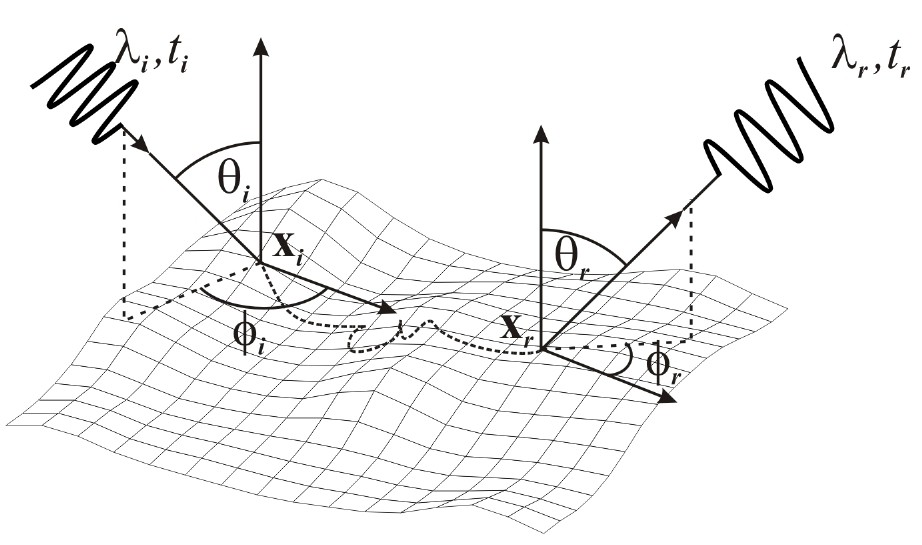The illumination on a given point of the surface depends on: The viewpoint The surface properties reflexion absorption diffusion The light properties direction wavelenght energy Involve a lot of dimensions

## Color

Physically, visible light is an electromagnetic wave

##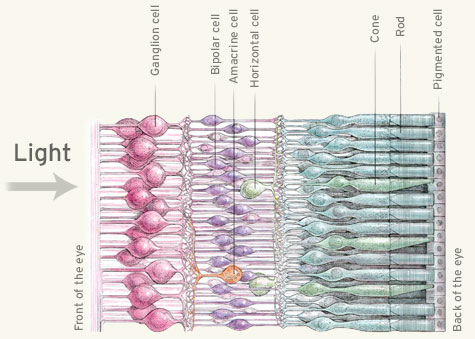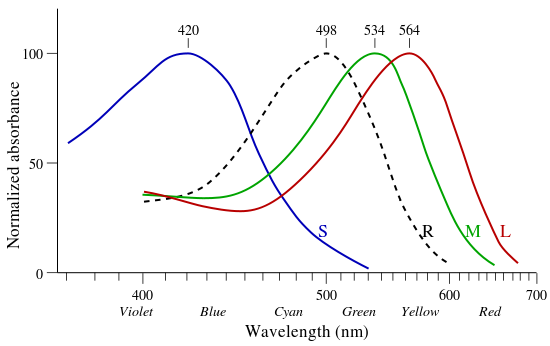Physiologically, our eyes use 3 types of sensors to perceive light colors

### In computer science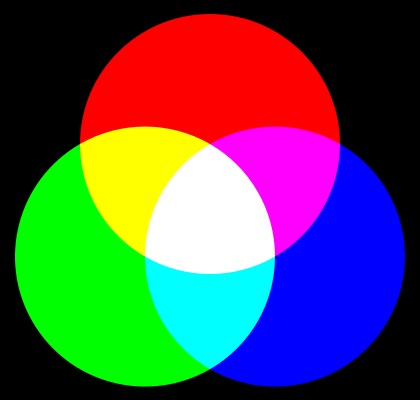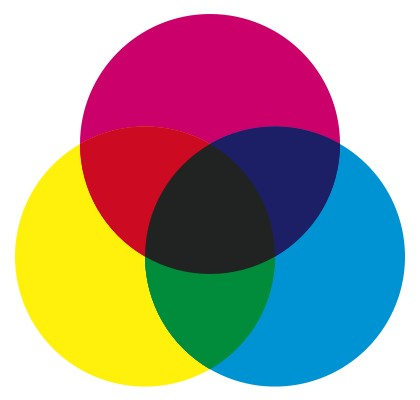Additive models (lighting) Soustractive models (painting)
• RGB(A) for Red, Green, Blue (Alpha) is the most well known additive model
• Easy to use in graphics applications
• Difficult to ensure the same color on different screen...  (gamut)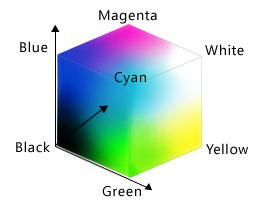• CIE 1931 XYZ color space
• The tristimulus values can be conceptualized as amounts of three primary colors in a trichromatic additive color model.
• The CIE (Comission Internationale de l'éclairage) was the first to mathematically define a trichromatic system to represent perceived colors.
• It is based on the standard (colorimetric) observer and thus allows to uniquely represent colors in a 3D space.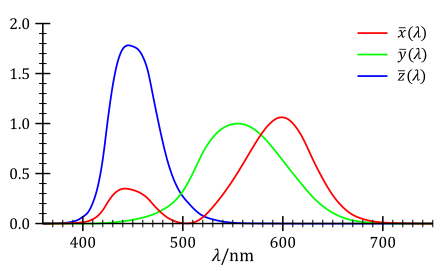Color matching functions: spectral sensitivity curves of 3 linear light detectors If $$I (\lambda)$$ is the spectral distribution of a color, then: $X = \int_{400}^{800} I(\lambda) \bar{x}(\lambda) d \lambda$ $Y = \int_{400}^{800} I(\lambda) \bar{y}(\lambda) d \lambda$ $Z = \int_{400}^{800} I(\lambda) \bar{z}(\lambda) d \lambda$
• CIE Yxy color space
• Normalize the XYZ color space and decompose it into Luminance (Y) and chrominance (xy)
• CIE Lab color space
• Perceptually uniform: distance between 2 colors = perceived distance between these colors
• Used in most graphics applications
• L = luminance
• a = chrominance (red-green)
• b = chrominance (blue-yellow)
• HSV (Hue, Saturation, Value) color space
• In most image processing software: Photoshop, Gimp, etc.
• Intuitive for designers
• CMY(K), for Cyan, Magenta, Yellow (and Key - Black)
• Subtractive model
• Mainly used for printing
• ETC....: Adobe RGB, sRGB, CIELuv, CIEUvw, YIQ (NTSC), YUV (PAL), HSL, ....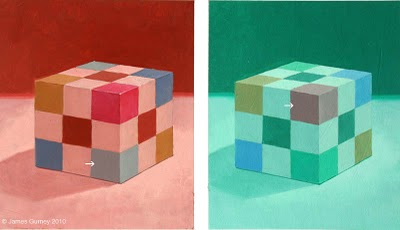Still, the perception of colors is not well understood and the perfect color space does not exist! (example of color contrast) try this excellent demo

### Coding colors

• Binary representation: 0 or 1
• 8 bits: 0 to 255 grey levels (monochromatic)
• 24 bits: 8 bits per channel (polychromatic)
• 256 values per channel (usually RGB)
• 256*256*256 = 16 777 216 colors
• limited: 8 orders of magnitude between the sun and stars
• HDR (High Dynamic Range) images: float or double precision per channel
• 24 bits per channel = $$256^9$$ colors
• Can be created using multiple LDR (Low Dynamic Range) images
• Needs to be tonemapped to be displayed on a screen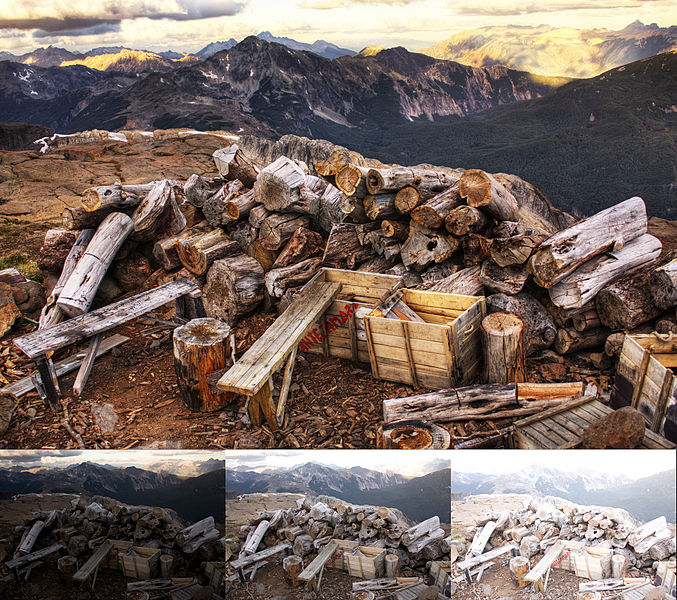## The rendering equation [Kajiya 1986]

$L(\mathbf{p} \rightarrow \mathbf{e}) = L_e(\mathbf{p} \rightarrow \mathbf{e}) + \int_{\Omega_\mathbf{n}} \rho(\mathbf{p}, \mathbf{e}, \pmb{\ell}) (\mathbf{n}\cdot\pmb{\ell}) \ L(\mathbf{p} \leftarrow \pmb{\ell}) \ d\pmb{\ell}$
• $$\mathbf{p}$$ is a point on the surface
• $$\mathbf{e}$$ is the view direction
• $$\mathbf{n}$$ is the normal of the surface at point $$\mathbf{p}$$
• $$\pmb{\ell}$$ is the direction of a light in the hemisphere $$\Omega_\mathbf{n}$$
• $$L(\mathbf{p} \rightarrow \mathbf{e})$$:
• outgoing radiance (in $$Wm^{-2}sr^{-1})$$
• how much energy is arriving to the eye / camera
• $$L_e(\mathbf{p} \rightarrow \mathbf{e})$$:
• usually equal to 0 for object surfaces (they do not create energy)
• $$L(\mathbf{p} \leftarrow \pmb{\ell})$$:
• incident illumination leaving the light $$\pmb{\ell}$$ and arriving at the point $$\mathbf{p}$$ of the surface
• $$(\mathbf{n}\cdot\pmb{\ell})$$:
• the orientation of the surface
• dot product between $$\mathbf{n}$$ and $$\pmb{\ell}$$
• $$\rho(\mathbf{p}, \mathbf{e}, \pmb{\ell})$$:
• material properties / BRDF (Bidirectional Reflectance Distribution Function)
• how much energy the surface reflects in the viewing direction $$\mathbf{e}$$ given the incident light $$\pmb{\ell}$$

## Local lighting

### General equation

Empirical model for computing the outgoing radiance
$L(\mathbf{p} \rightarrow \mathbf{e}) = \rho_a L_a + \sum_{k} \rho(\mathbf{p}, \mathbf{e}, \pmb{\ell}_k) \ (\mathbf{n}\cdot\pmb{\ell}_k) \ L(\mathbf{p} \leftarrow \pmb{\ell}_k)$

• $$L(\mathbf{p} \rightarrow \mathbf{e})$$: outgoing radiance / light energy / color
• $$\rho_a L_a$$: ambient lighting (approximate indirect lighting)
• $$\sum_{k} \cdots$$: contribution of each light $$\pmb{\ell}_k$$
• $$\rho(\mathbf{p}, \mathbf{e}, \pmb{\ell}_k)$$: BRDF - how the light $$\pmb{\ell}_k$$ is reflected on top of the surface
• $$(\mathbf{n}\cdot\pmb{\ell}_k)$$: surface orientation (according to light $$\pmb{\ell}_k$$ )
• $$L(\mathbf{p} \leftarrow \pmb{\ell}_k)$$: incoming radiance for light $$\pmb{\ell}_k$$

### The simplest model: assign a color at each point of the surface (albedo)

$L(\mathbf{p} \rightarrow \mathbf{e}) = color$
• Reflecting power of the surface
• Independent of the view direction
• Independent of the light direction
• Too simple:
• adapted for static scenes (photos) but not for dynamic ones (image synthesis)
• dynamic viewpoint?
• dynamic lighting variations?

### The second simplest model: consider a single dynamic light

$L(\mathbf{p} \rightarrow \mathbf{e}) = \rho(\mathbf{p}, \mathbf{e}, \pmb{\ell}) \ (\mathbf{n}\cdot\pmb{\ell}) \ L(\mathbf{p} \leftarrow \pmb{\ell})$
• Which types of lights?
• Which types of surface reflections?

## Types of lights

Infinitesimal lights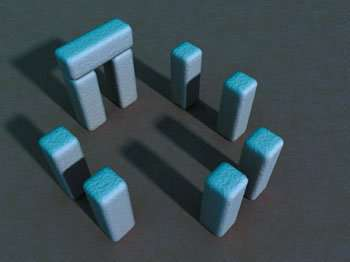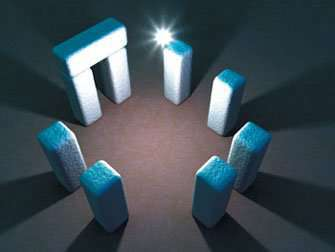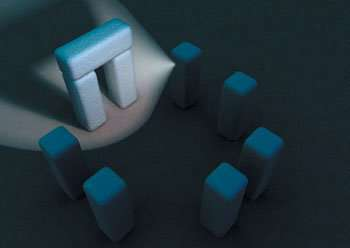Directionnal light Distant sources (sun) Environment maps (video-games) $L(\mathbf{p} \leftarrow \pmb{\ell}) = L$ Point light position in space $$\mathbf{p}_{\pmb{\ell}}$$ near, small sources $L(\mathbf{p} \leftarrow \pmb{\ell}) = L/r^2$ with $$r = || \mathbf{p} - \mathbf{p}_{\pmb{\ell}} ||$$ and $$\pmb{\ell} = \frac{\mathbf{p} - \mathbf{p}_{\pmb{\ell}} }{r}$$ Spot light position in space near, small sources defined in a cone direction $$\mathbf{s}_{\pmb{\ell}}$$ exponent $$e$$ a cutoff $$c$$ $L(\mathbf{p} \leftarrow \pmb{\ell}) = \frac{(\mathbf{s}_{\pmb{\ell}} \cdot \pmb{\ell})^e L}{r^2}$ if $$(\mathbf{s}_{\pmb{\ell}} \cdot \pmb{\ell}) Area lights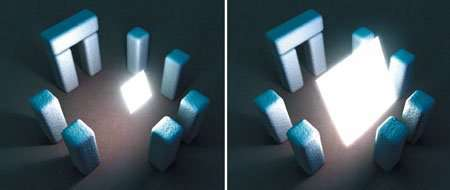Defined on volumes / surfaces Soft shadows Expensive! ## Types of reflections Lets consider a (white) directional light ( \( L=cst=(1,1,1)$$ ). The model is equal to:
$L(\mathbf{p} \rightarrow \mathbf{e}) = \rho(\mathbf{p}, \mathbf{e}, \pmb{\ell}) \ (\mathbf{n}\cdot\pmb{\ell})$

### The lambertian model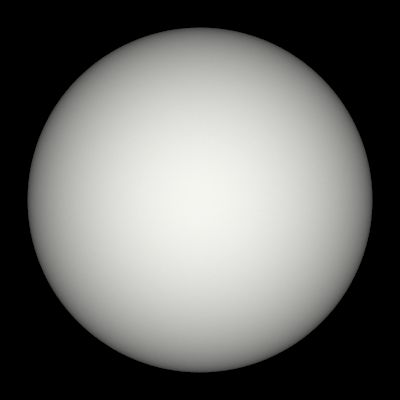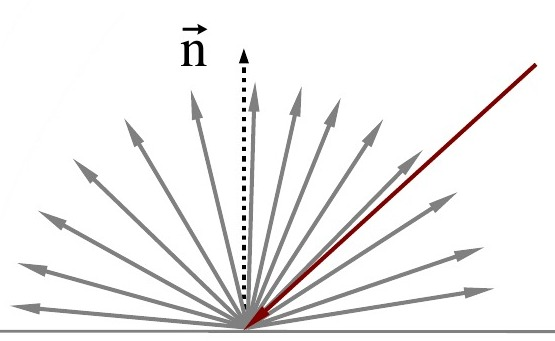Lambertian surface $\rho(\mathbf{p}, \mathbf{e}, \pmb{\ell}) = cst$ Light is diffused in every direction Independant on the point of view

 $L(\mathbf{p} \rightarrow \mathbf{e}) = \rho_d \ (\mathbf{n}\cdot\pmb{\ell}) L_d$ $L(\mathbf{p} \rightarrow \mathbf{e}) = \sum_k \rho_{kd} \ (\mathbf{n}\cdot\pmb{\ell}) L_{kd}$ One light: $$\rho_d =$$ constant diffuse material color  $$L_d =$$ constant diffuse light color Multiple light sum of contribution of each light may have different color coeficients

### The mirror and transparent models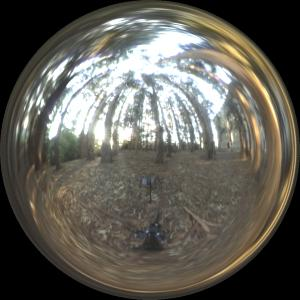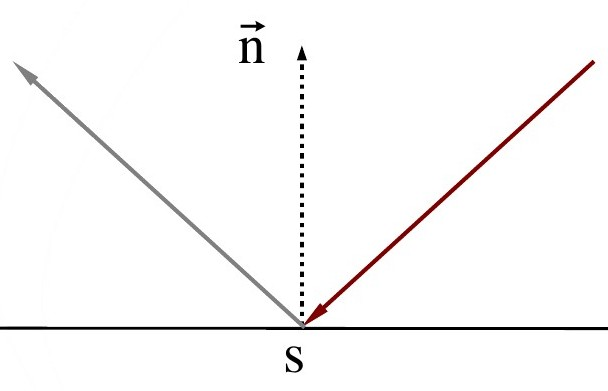Mirror surface $\rho(\mathbf{p}, \mathbf{e}, \pmb{\ell}) = cst\ if\ (\mathbf{r}\cdot\mathbf{e}=1),\ 0\ otherwise$ Reflected light vector $$\mathbf{r}=2 \mathbf{n} (\mathbf{n}\cdot \pmb{\ell})-\pmb{\ell}$$ Dependant on the point of view Usefull for environment maps compute the reflected view vector $$\mathbf{r}=2 \mathbf{n} (\mathbf{n}\cdot \mathbf{e})-\mathbf{e}$$ use it to fetch a color in the map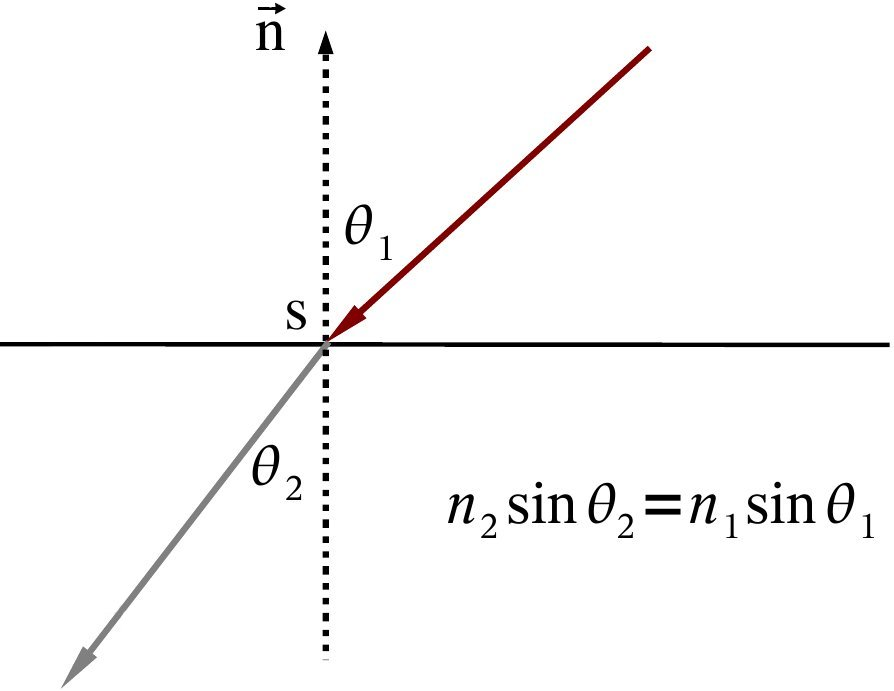Transparent surface $\rho(\mathbf{p}, \mathbf{e}, \pmb{\ell}) = cst\ if\ (\mathbf{r}\cdot\mathbf{e}=1),\ 0\ otherwise$ Refracted light vector $$\mathbf{r}=e \pmb{\ell} - (e (\mathbf{n}\cdot \pmb{\ell}) + \sqrt{1-e^2 (1-(\mathbf{n}\cdot \pmb{\ell})^2)}) \mathbf{n}$$ Dependant on the point of view Same principle for environment maps

 $L(\mathbf{p} \rightarrow \mathbf{e}) = \rho_s(\mathbf{p}, \mathbf{e}, \pmb{\ell}) \ (\mathbf{n}\cdot\pmb{\ell}) L_s$ $L(\mathbf{p} \rightarrow \mathbf{e}) = \sum_k \rho_{ks} (\mathbf{p}, \mathbf{e}, \pmb{\ell})\ (\mathbf{n}\cdot\pmb{\ell}) L_{ks}$ One light: $$\rho_s =$$ constant specular material color  $$L_s =$$ constant specular light color Multiple light sum of contribution of each light may have different color coeficients

### Glossy models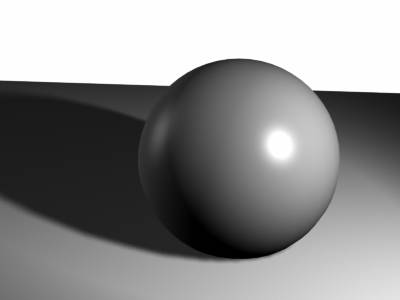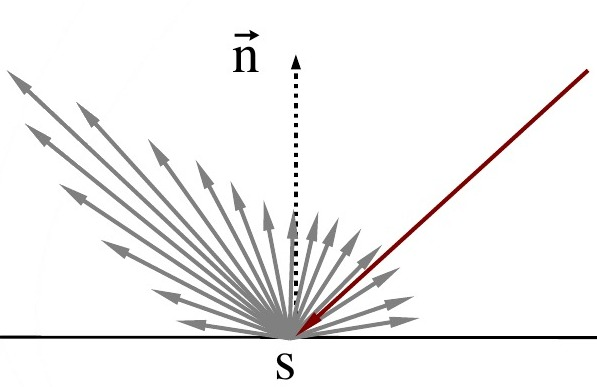Glossy surface $\rho(\mathbf{p}, \mathbf{e}, \pmb{\ell}) = \rho_d(\mathbf{p}, \mathbf{e}, \pmb{\ell}) + \rho_s(\mathbf{p}, \mathbf{e}, \pmb{\ell})$ Usually expressed as a sum of diffuse and specular terms $$\rho_d = cst$$ (as before) $$\rho_s = (\mathbf{r}\cdot\mathbf{e})^e$$ (for instance)

### The Phong model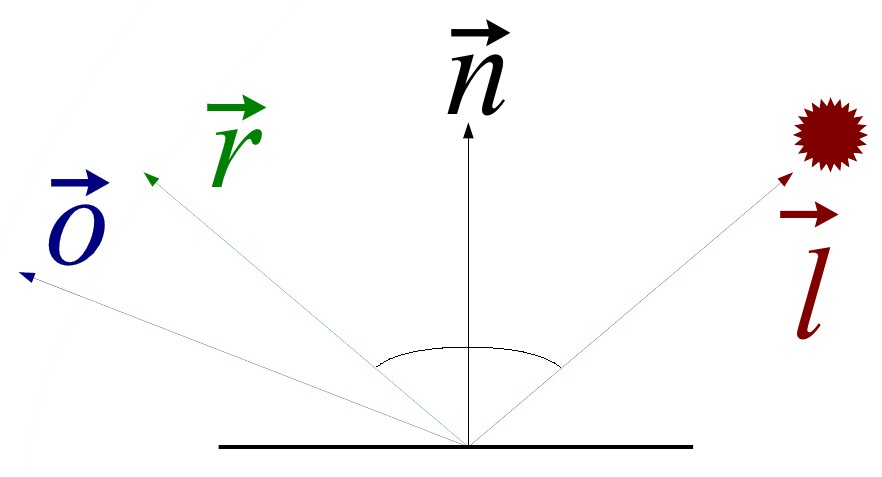$$\rho_s = (\mathbf{r}\cdot\mathbf{e})^e$$

The general formulation of the Phong model is given by a weighted sum of an ambient, diffuse and specular term:
$L(\mathbf{p} \rightarrow \mathbf{e}) = \rho_a L_a + \sum_k \rho_{kd} L_{kd} (\mathbf{n}\cdot\pmb{\ell}) + \rho_{ks} L_{ks} (\mathbf{r}\cdot\mathbf{e})^e$
where
• $$\rho_a$$, $$\rho_{kd}$$ and $$\rho_{ks}$$ are the material colors for the ambient, diffuse and specular term, respectively.
• $$L_a$$, $$L_{kd}$$ and $$L_{ks}$$ are the light colors for the ambient, diffuse and specular term, respectively.### The Blinn-Phong Model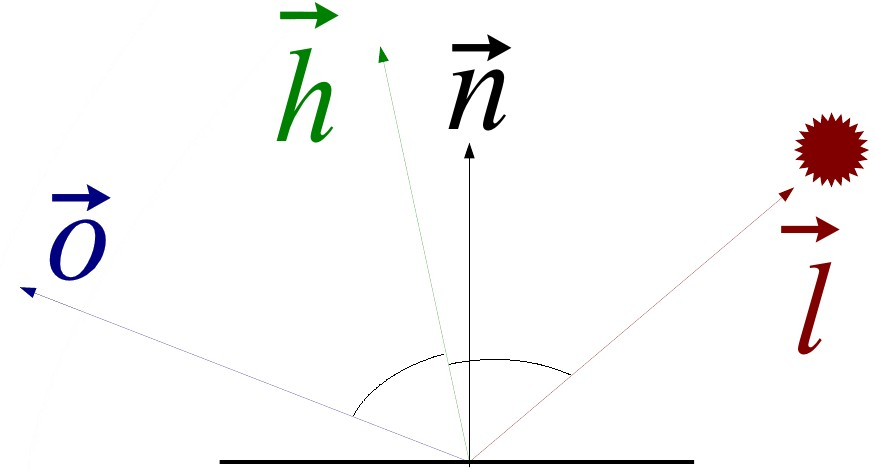Specular term replaced by $$\rho_s = (\mathbf{h}\cdot\mathbf{n})^e$$ , with $$\mathbf{h}=\frac{\pmb{\ell}+\mathbf{e}}{|| \pmb{\ell}+\mathbf{e}||}$$

### Anisotropy effect

Specular term replaced by $$\rho_s = (\mathbf{h}\cdot\mathbf{n})^{n_u cos^2\phi + n_v sin^2 \phi}$$

### Fresnel effect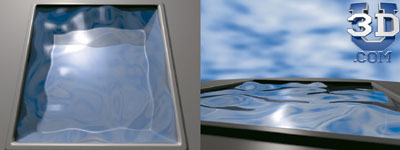Obtained using Schlick approximation: $$F = R_s + (1-R_s)(1-\mathbf{e}\cdot\mathbf{h})^5$$

### Other effects?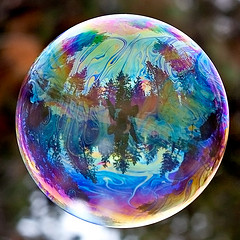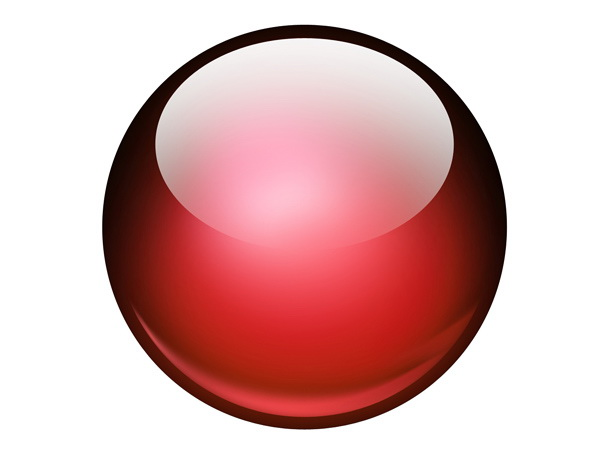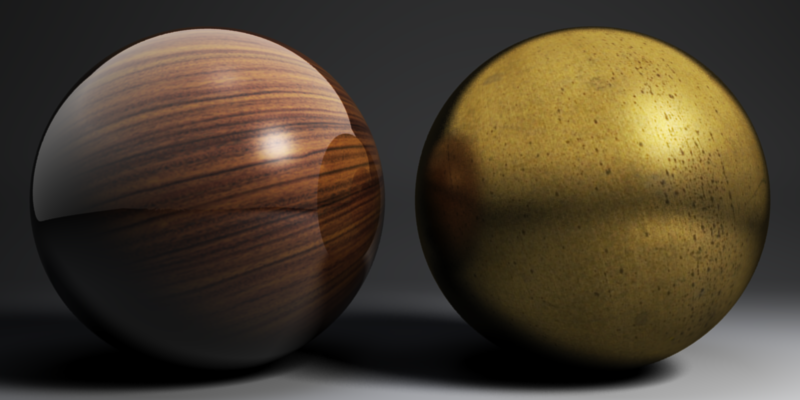Diffraction Non-realistic effects Varying material properties
• Plenty of BRDFs (they are specialized for certain kinds of materials)
• Lambertian
• Phong
• Blinn-Phong
• Torrance-Sparrow
• Cook-Terrance
• Ward
• Oren-Nayar
• Ashikhmin-Shirley
• Lafortune
• etc...
• And also SVBRDF (for spatially varying BRDF)
• material parameters change over the surface (usually using textures
• And also BSSRDF (for Bidirectionnal Subsurface Scatering Distribution Function)
• specific for translucent materials
• More generally: BXDF for Bidirectionnal X Distribution Function

## How to compute lighting on the GPU?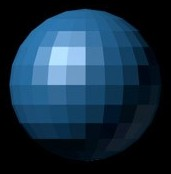Compute lighting per face In the vertex shader Normals are constant for the vertices of each triangle Produce shading discontinuities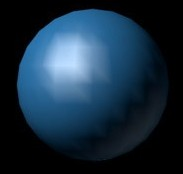Compute lighting per vertex In the vertex shader Normals are different for each vertex Color is computed and interpolated during rasterization Quality / result depends on tesselation Question: what is the color at point M?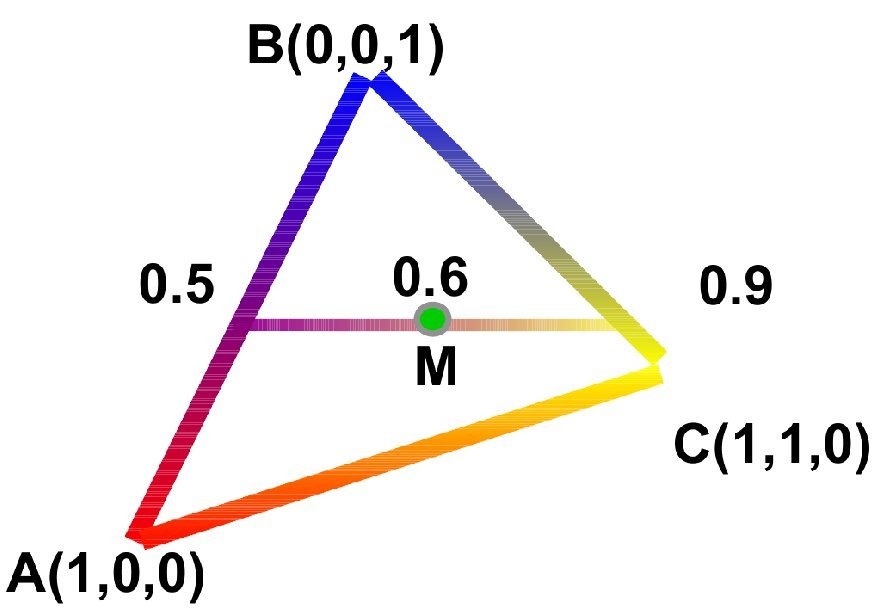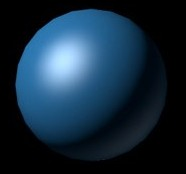Compute lighting per fragment Normals are interpolated during rasterization (vertex to fragment) Normals are re-normalized Color is computed Best result